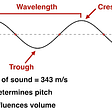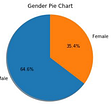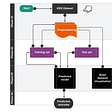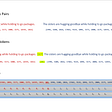# How To Handle Unbalanced Dataset.

Indeed , unbalanced data set is biggest challengeable task in machine learning.
It’s common problem in machine learning specially in classification.
It effect on model accuracy and lead overfitting.

There is some domain. where handle unbalanced data is biggest challengeable task.
1-Spam classification problem.
2-Diseases screening.
3-Fraud detection.
etc.

In all these domains majority of data belong to a single particular class.

Right here , we will discuss How to deal with unbalanced dataset and what will be approach to handle unbalanced dataset.

1. We will consider simple dataset which is spam classifier and we will see what is the proposition of classes along accuracy of the model with unbalanced dataset and then we will see accuracy using confusion matrix. how all prediction belong to a single particular class.

2. We will see some basic techniques which is mostly useful to handle unbalanced data.

First check the model accuracy and how all predicted class belong to a single particular class.

STEP-1

`import pandas as pddata=pd.read_csv('spam.csv',encoding='ISO-8859-1')data.rename(columns={'v1':'Target','v2':'Text'},inplace=True)target_count = data.Target.value_counts()print('Class 0:', target_count)print('Class 1:', target_count)print('Proportion:', round(target_count / target_count, 2), ': 1')`

Here, proportion of classes.
You can see, it is totally unbalanced data with 6.46 : 1 proportion.
Let see with the graph.

`target_count.plot(kind='bar', title='Count (target)');`

Majority of the data belong to ham class.

Let check, what accuracy and confusing matrix is saying about unbalanced data.

STEP 2-

`data=data.drop(['Unnamed: 2','Unnamed: 3','Unnamed: 4'], axis=1)dataset=data.copy()from sklearn import preprocessinglabel_encoder = preprocessing.LabelEncoder()data['Target']= label_encoder.fit_transform(data['Target'])data_sen=data['Text']output_variable=data['Target']import nltkimport refrom nltk.corpus import stopwordsfrom nltk.stem import WordNetLemmatizernltk.download('stopwords')after_preprocessing_of_text=[]Lemmatizer = WordNetLemmatizer()nltk.download('wordnet')for i in range(0, len(data_sen)):   rev = re.sub('[^a-zA-Z]', ' ', data_sen[i])   rev = rev.lower()   rev = rev.split()   rev = [Lemmatizer.lemmatize(word) for word in rev if not word in           stopwords.words('english')]   rev = ' '.join(rev)   after_preprocessing_of_text.append(rev)from sklearn.feature_extraction.text import TfidfVectorizerTF_IDF_tech = TfidfVectorizer()X = TF_IDF_tech.fit_transform(after_preprocessing_of_text).toarray()from sklearn.metrics import confusion_matrixfrom sklearn.linear_model import LogisticRegressionourmodel = LogisticRegression(solver = 'lbfgs')from sklearn.model_selection import train_test_splitX_train, X_test, y_train, y_test = train_test_split(X, output_variable, test_size=0.3, random_state=1)ourmodel.fit(X_train,y_train)y_pred = ourmodel.predict(X_test)from sklearn import metricsaccuracy = metrics.accuracy_score(y_test, y_pred)print('Accuracy: {:.2f}'.format(accuracy))`

Accuracy of logistics regression is very good with this unbalanced data 96%.

An interesting way to evaluate the results is by means of a confusion matrix, which shows the correct and incorrect predictions for each class. In the first row, the first column indicates how many classes 0 were predicted correctly, and the second column, how many classes 0 were predicted as 1. In the second row, we note that all class 1 entries were erroneously predicted as class 0.

Therefore, the higher the diagonal values of the confusion matrix the better, indicating many correct predictions.

`from sklearn.metrics import confusion_matrixfrom matplotlib import pyplot as pltconf_mat = confusion_matrix(y_true=y_test, y_pred=y_pred)print('Confusion matrix:\n', conf_mat)labels = ['Class 0', 'Class 1']fig = plt.figure()ax = fig.add_subplot(111)cax = ax.matshow(conf_mat, cmap=plt.cm.Blues)fig.colorbar(cax)ax.set_xticklabels([''] + labels)ax.set_yticklabels([''] + labels)plt.xlabel('Predicted')plt.ylabel('Expected')plt.show()`

But majority of predict belong to ham class. it might be possible it leading overfitting.

Handle the unbalanced dataset — -

Resampling — — -

A widely adopted technique for dealing with highly unbalanced datasets is called resampling. It consists of removing samples from the majority class (under-sampling) and / or adding more examples from the minority class (over-sampling).

Despite the advantage of balancing classes, these techniques also have their weaknesses . The simplest implementation of over-sampling is to duplicate random records from the minority class, which can cause overfitting. In under-sampling, the simplest technique involves removing random records from the majority class, which can cause loss of information.

Let’s implement a basic example, which uses the DataFrame.sample method to get random samples each class:

STEP 3- Random under sampling

`count_class_0, count_class_1 = data.Target.value_counts()target_class_0 = data[data['Target'] == 0]target_class_1 = data[data['Target'] == 1]`

How its work you had 747 is spam messages and 4825 is ham messages. so its unbalance dataset , for do balance we are applying under-sampling. you need to know some important points before apply under-sampling.

1. If data set is large . you can perform under-sampling. like in this case here dataset is large so here we are applying under sampling.
2. Under-sampling work like — if you have dataset 1000 rows , 100 yes rows or 900 no rows. so its totally unbalance dataset. so what will happen, when you use under sampling. under-sampling count how many records is yes total 100 yes . then under-sampling pick random 100 records from no data points . and then after performing under-sampling your new dataset with 100 yes or 100 no. then that data you can perform any machine learning. whichever you like. but, your dataset is not large then go for oversampling.
`data_class_0_under = target_class_0.sample(count_class_1)data_test_under = pd.concat([data_class_0_under, target_class_1], axis=0)print('Random under-sampling:')print(data_test_under.Target.value_counts())data_test_under.Target.value_counts().plot(kind='bar', title='Count (target)');`

Right here, 747 samples random picked up from ham which represent with 0 .
Total ham data -4825( randomly picked 747 data point from ham class by under-sampling)
Total spam data — 747
Total data is 747+747 with equal ratio.
So, data is balanced using under-sampling.

STEP 4- Random oversampling

How oversampling is work?

Its opposite of under-sampling. in under-sampling reduce the size but in oversampling increase the size. . when you implement nd use oversampling , some important points you have to remember-

1. It use for small dataset

2. If you have 100 dataset , which 10 is yes or 90 is no

So according to oversampling

no data will be create , then after perform oversampling

Our new dataset is 90 is yes 90 is no , total dataset is 180.

Random oversampling using sample method.

`data_class_1_over = target_class_1.sample(count_class_0, replace=True)data_test_over = pd.concat([target_class_0, data_class_1_over], axis=0)print('Random over-sampling:')print(data_test_over.Target.value_counts())data_test_over.Target.value_counts().plot(kind='bar', title='Count (target)');`

In oversampling 4078 Data points created of 1 class which is spam by over-sampler .
Total ham data point -4825
Total spam data point -747 + 4078 = 4825.
Oversampling create data and balance the dataset.

Now data is balanced using oversampling.

Now let see resampling using imblearn python library.

Let’s apply some of these resampling techniques, using the Python library imbalanced-learn. It is compatible with scikit-learn and is part of scikit-learn-contrib projects.

Random oversampling using imblearn library

`from imblearn.over_sampling import RandomOverSampler os =  RandomOverSampler(ratio=1) `

#ratio is the power of this . if ratio is 1 mean we create 50 -50 dataset

# if ratio is 0.5 mean we create 75–25 ratio dataset.

# example — if your dataset is yes 500 or No is 100 and you select ratio=1 , it mean 400 new data set will create of No.

# so total no is 100 + 400 =500 . so yes is 500 , No also be 500 . then your dataset is 50–50

# if your dataset is yes 500 or No 100 and you select ratio=0.5 , it mean 150 new dataset will be create of No

#so total no is 100+150 =250 . so your dataset ratio is 500 yes or 250 No.

# so here ratio play important role.

# if you want specific particular ratio of dataset you can use it.

`labels = dataset.columns[1:]Xx=dataset[labels]Yy=dataset["Target"]X_train_res, y_train_res = os.fit_sample(Xx, Yy)from collections import Counterprint('Original dataset shape {}'.format(Counter(Yy)))print('Resampled dataset shape {}'.format(Counter(y_train_res)))`

You can see before resampling our dataset is ham-4825 and spam — 747.

After performed over-sampling using imblearn library. Dataset became balanced with same no of ham and same no of spam.

No majority of data belong to single class after performed resampled.

`from imblearn.under_sampling import RandomUnderSampleroverr = RandomUnderSampler(return_indices=True)X_overr, y_overr, id_overr = overr.fit_sample(Xx, Yy)print('Original dataset shape {}'.format(Counter(Yy)))print('Resampled dataset shape {}'.format(Counter(y_overr)))`

You can see before resampling our dataset is ham-4825 and spam — 747.

After performed Under-sampling using imblearn library. Dataset became balanced with same no of ham and same no of spam.

No majority of data belong to single class after performed resampled.

Now your data is balanced . you can perform any Algorithm. because majority of data not belong to single class.

--

--

--

## More from Puneet Singh

Data Science , Machine Learning , BlockChain Developer

Love podcasts or audiobooks? Learn on the go with our new app.

## Acoustic machine learning series: Recorded sounds into AI/ML algorithms## Talking Data User Demographics## Math behind GBM and XGBoost## Video-Language Pre-training based on Transformer Models## Exploring the Patterns in Brain Signals: State-of-the-Art of Machine Learning## Artificial Neural Network (ANN) in the Classification of Iris Species Using R-Studio## Why we should be Deeply Suspicious of BackPropagation## Puneet Singh

Data Science , Machine Learning , BlockChain Developer

## Iris Dataset, But Make It Interesting!## Multiclass classification for Natural Language Inference## Display Prediction Probabilities of Multiclass Classification Using Bar Chart